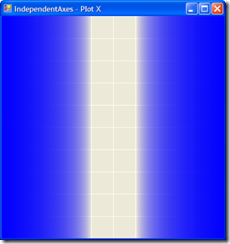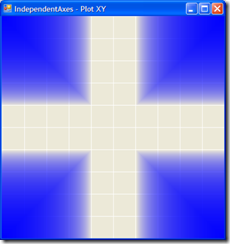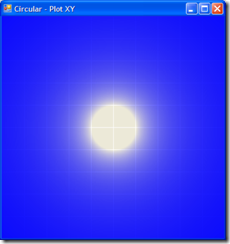## Monday, April 30, 2007

The last couple of nights I've been working on the dead zone functionality for the GamePad class in MonoXna, which was an interesting excursion off into formula's and equations I haven't been exposed to for many years.

I read an post by Shawn Hargreaves, which gave me a good start on understanding the MSXNA implementation.  I've now implemented the basic functionality of the two algorithms, IndependentAxis and Circular.

I used Shawn's formula for the IndependentAxis deadzone calculation and here is a plot of this:

 Independent Axis - Plot X Independent Axis - Plot XYI ended up using a variation of Shawn's formula for the IndependentAxis and using a bit of trig, ended up with the following for the Circular implementation:

```/// <summary>
/// As per http://blogs.msdn.com/shawnhar/archive/2007/03/28/gamepads-suck.aspx
/// Both <paramref name="x"/> and <paramref name="y"/> parameters assumed to be between -1.0 and 1.0
/// </summary>
static Vector2 CircularAxisDeadZone(float x, float y)
{
float dist = (float)Math.Max(Math.Sqrt(x * x + y * y), EPSILON);
 Circular - Plot XY